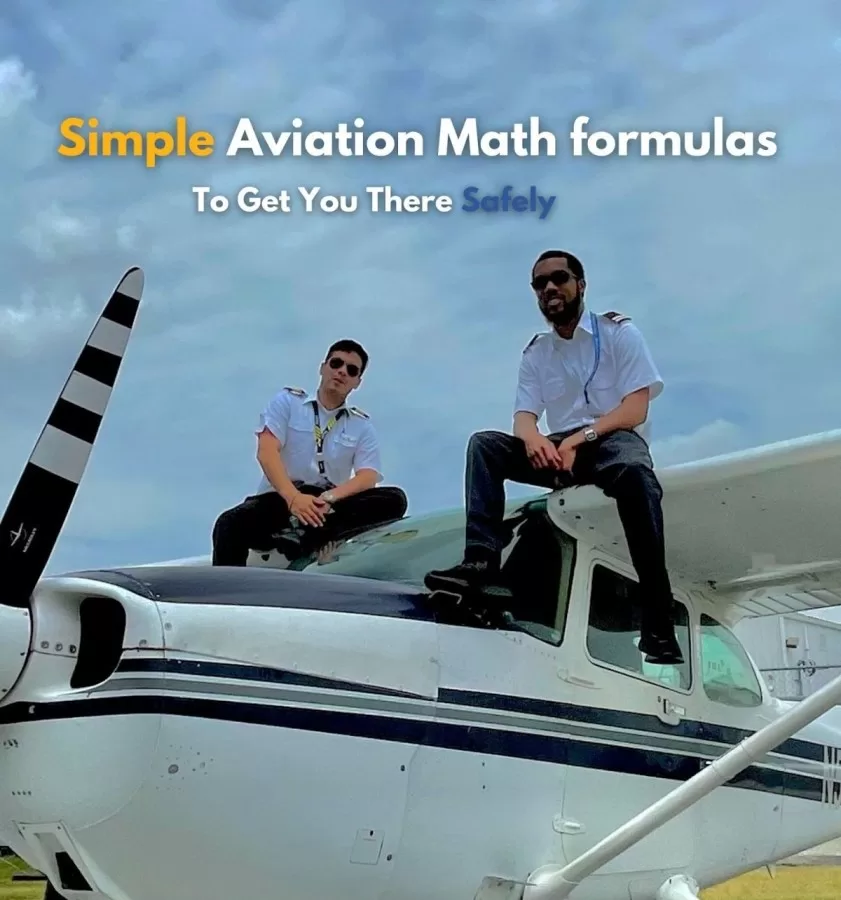# Mastering The Math Of Flight Simple Aviation Formulas You Need To KnowAviation is a fascinating field that involves flying, maintaining, and operating aircraft. Pilots, technicians, and engineers use a variety of math formulas in their daily work. From calculating fuel consumption to estimating the weight of passengers and cargo, math plays a crucial role in aviation. In this article, we will explore some simple aviation math formulas that every aspiring aviation professional should know.

Wayman Aviation Academy,  is the ideal flight school in Florida for those seeking exceptional flight training courses. With a comprehensive range of training programs catering to domestic and international students, this flight school is a popular choice for aspiring pilots and those looking to enhance their flying skills. Whether you are interested in starting your career as a pilot or simply want to gain knowledge about aviation, Wayman Aviation Academy offers a plethora of free resources and information to help you succeed in your career journey. Head over to their website today and take advantage of their invaluable resources.

## Distance, Speed, and Time

One of the most fundamental aviation math formulas is the Distance, Speed, and Time formula (DST). It is used to calculate the time taken to travel a certain distance at a specific speed. The formula is:

Time = Distance / Speed

For example, if a plane travels 500 miles at a speed of 250 miles per hour, the time taken would be:

Time = 500 / 250 = 2 hours

## Fuel Consumption

Another important aviation math formula is fuel consumption. Knowing how much fuel a plane needs for a particular flight is crucial for safety and efficiency. The formula for fuel consumption is:

Fuel consumption = (Fuel flow rate x Time) / Distance

For example, if a plane burns 100 gallons of fuel per hour and travels 500 miles in 2 hours, the fuel consumption would be:

Fuel consumption = (100 x 2) / 500 = 0.4 gallons per mile

## Weight and Balance

Maintaining proper weight and balance is critical for the safe operation of an aircraft. The weight and balance formula is used to calculate the total weight of the aircraft, including passengers, cargo, and fuel. The formula is:

Total weight = Empty weight + Payload weight

where the empty weight is the weight of the aircraft without any passengers, cargo, or fuel, and the payload weight is the weight of passengers, cargo, and fuel. It is also essential to ensure that the weight is distributed evenly throughout the aircraft. The center of gravity (CG) must be within the allowable limits specified in the aircraft’s manual. The formula for CG is:

CG = (Moment / Total weight)

where the moment is the product of the weight and the distance from the reference point (usually the nose of the aircraft).

## Temperature Conversion

Aviation professionals must be able to convert between Celsius and Fahrenheit temperatures. The formulas for temperature conversion are:

Fahrenheit to Celsius: C = (F – 32) x 5/9

Celsius to Fahrenheit: F = (C x 9/5) + 32

For example, if the temperature is 68 degrees Fahrenheit, the equivalent Celsius temperature would be:

C = (68 – 32) x 5/9 = 20 degrees Celsius

## Altitude and Air Pressure

At high altitudes, the air pressure decreases, and it is essential to know the altitude and the corresponding air pressure. The formula for calculating air pressure is:

Pressure = (Density x Acceleration due to gravity x Altitude)

where the density is the mass per unit volume of air, and the acceleration due to gravity is 9.8 meters per second squared. At sea level, the standard air pressure is 1013.25 hectopascals (hPa). For every 1000 feet of altitude gained, the air pressure decreases by approximately one inch of mercury (inHg).

## Mach Number

The Mach number is a dimensionless quantity that represents the speed of an aircraft relative to the speed of sound. It is a critical parameter for high-speed flight. The formula for calculating Mach number is:

Mach number = True airspeed / Speed of sound

The speed of sound varies with temperature and altitude. At sea level and 59 degrees Fahrenheit, the speed of sound is 761 miles per hour.

In conclusion, aviation math formulas are crucial in the aviation industry. They help Commercial pilots, technicians, and engineers to ensure the safety and efficiency of aircraft operations. The formulas discussed in this article are simple and fundamental, but they are just the tip of the iceberg. There are numerous other math formulas used in aviation, such as those related to navigation, weather, and performance. Aspiring aviation professionals should strive to develop a strong foundation in mathematics to excel in this exciting field. To find out more in-depth info about these aviation math formulas you can visit Wayman’s blog. Get more details and explanations.